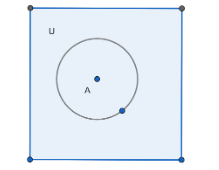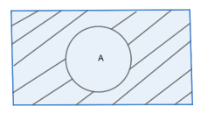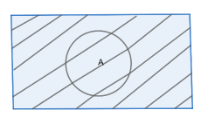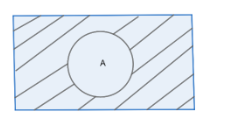QuestionAnswers

# If $A\subset U$ , prove that:(A). U’ = $\phi$ (B). $\phi$’ = U(C). (A’)’ = A(D). $A\cup A'=U$ (E). $A\cap A'=\phi$Hint: First we will draw the Venn diagram with universal set U and subset A. Then we will solve this question by understanding the meaning of complement of a set, and then what is union and intersection of sets. We can check each option by trying to relate the concepts and find the sets. Then, we will be able to prove all the given options.

Universal set: The set containing all objects or elements and of which all other sets are subsets.
Complement of a set: Complement of a set A, denoted by A c, is the set of all elements that belongs to the universal set but does not belong to set A.
Union: The union (denoted by $\cup$ ) of a collection of sets is the set of all elements in the collection. It is one of the fundamental operations through which sets can be combined and related to each other.
Intersection: The intersection of two sets has only the elements common to both sets. If an element is in just one set it is not part of the intersection. The symbol is an upside down $\cap$ .Here U is the universal set which is the area of total square and circle is the set A.
Let’s solve (a).
From this diagram we can see that U’ means the elements that are not present in U.
Hence, we get $U'=U-U=\phi$
Hence, it will be empty set which is $\phi$ .
Hence we have proved (a).
Now we will solve part (b).
$\phi '=U-\phi$
But $\phi$ is an empty set.
Therefore, $\phi '=U$ as the complement of empty set is universal set.
Hence (b) has been proved.
Now, for (c)
A’ = U – A
Now again taking complement,
(A’)’ = U – A’ = A
Hence we have proved (A’)’ = A.
From the diagram also we can see that it is true.
Now we will solve (d),
First we will look at A’,Now the Venn diagram of $A\cup A'$ is:From the diagram and definition of union we can see that $A\cup A'=U$.
Hence we have proved (d).
Now we will prove (e),
First we will look at A’,From the diagram and definition of intersection we can see that $A\cap A'=\phi$.
Hence proved (e).

Note: Here we have used the definition of the given terms and also the Venn diagram to prove the above statements. Venn diagrams are very helpful to solve these types of questions. We must solve this question carefully using the concepts correctly instead of just proving them somehow. So, it is necessary to draw a Venn diagram for each option, apply the concepts and then prove them. We must not get confused between concepts of the union and intersection of sets.

Functions of vitamin A, B, C, D, EThe Difference Between an Animal that is A Regulator and One that is A ConformerArea of a Sector of a Circle FormulaWhere There is a Will There is a Way EssayTransistor as a DeviceWater - A Wonder LiquidArea of a Rhombus FormulaPerimeter of a Trapezoid FormulaReflection on A Plane MirrorMutation - A Genetic ChangeImportant Questions for CBSE Class 6 English A Pact with The Sun Chapter 10 - A Strange Wrestling MatchImportant Questions for CBSE Class 6 English A Pact with The Sun Chapter 8 - A Pact with the SunImportant Questions for CBSE Class 6 English A Pact with The Sun Chapter 1 - A Tale of Two BirdsCBSE Class 7 Science Water: A Precious Resource WorksheetsImportant Questions for CBSE Class 11 Physics Chapter 4 - Motion in a PlaneImportant Questions for CBSE Class 6 English A Pact with The SunImportant Questions for CBSE Class 6 English Honeysuckle Chapter 8 - A Game of ChanceImportant Questions for CBSE Class 11 Business Studies Chapter 7 - Formation of a CompanyImportant Questions for CBSE Class 8 English Honeydew Chapter 7 - A Visit to CambridgeImportant Questions for CBSE Class 7 Science Chapter 16 - Water: A Precious ResourceCBSE Class 10 Hindi A Question Paper 2020Hindi A Class 10 CBSE Question Paper 2009Hindi A Class 10 CBSE Question Paper 2015Hindi A Class 10 CBSE Question Paper 2016Hindi A Class 10 CBSE Question Paper 2012Hindi A Class 10 CBSE Question Paper 2010Hindi A Class 10 CBSE Question Paper 2008Hindi A Class 10 CBSE Question Paper 2014Hindi A Class 10 CBSE Question Paper 2007Hindi A Class 10 CBSE Question Paper 2013RS Aggarwal Class 8 Mathematics Solutions for Chapter-18 Area of a Trapezium and a PolygonNCERT Solutions for Class 12 English Kaliedoscope Poetry Chapter 4 Kubla Khan or a Vision in a Dream a FragmentNCERT Solutions for Class 6 English Honeysuckle Poem - A House, A HomeNCERT Solutions for Class 9 English Moments Chapter 8 - A House is Not a HomeNCERT Solutions for Class 12 Accountancy Chapter 3 - Reconstitution of a Partnership Firm: Admission of a PartnerNCERT Solutions for Class 12 Accountancy Chapter 4 - Reconstitution of a Partnership Firm:Retirement/Death of a PartnerRS Aggarwal Solutions Class 8 Chapter-18 Area of a Trapezium and a Polygon (Ex 18B) Exercise 18.2NCERT Solutions for Class 10 English Footprints Without Feet Chapter 4 - A Question of TrustRS Aggarwal Solutions Class 8 Chapter-18 Area of a Trapezium and a Polygon (Ex 18C) Exercise 18.3NCERT Solutions for Class 6 English A pact with the Sun Chapter-10